# Numeral Systems and Numbers Conversion in SQL

Numeral systems can be fascinating. In everyday programming, we are now becoming quite insulated from the need to convert between binary numbers and their representation, so it is a novelty to try out ways of doing it in SQL, and experiment with other number systems from the past.

My fascination with numbers started when I was about two years old.

One of my parents used to bend my fingers, saying “One, Two, Three…” and all our guests smiled, when I  tried to repeat “Four, Five, Six…”

At that time, I believed that people need the numbers in order to count their fingers and to make their parents and relatives laugh.

When I grew up, I discovered that numbers could be helpful in a few more situations, for example to identify time and count money. However, I did not pay much attention to the fact that the counting rules for time and money are different.

In one day, among the old documents, stored in our family, I found my grandfather’s watch. The watch had a beautiful watch face with strange symbols on it, instead of numbers. My mother told me that these symbols are the Roman numbers.

That fact, however, did not make me curious about the Roman numbers. In my mind, they were associated with the old, broken watch – both, the numbers and the watch were not in use.

I got my first hints of other numeral system at university.

The computers were built of transistors – small electronic devices with two physical states: opened and closed. These states, being coded as 0 and 1, perfectly fitted into binary numeral system. Based on that system and supported by the powerful mathematical apparatus, the computers changed human life.

If you carefully look around, you will find many signs of different numeral systems. Some of them are not relevant anymore; the other ones we still use and develop. Time, and angles, for example, are still based on sexagesimal systems.

### A Brief History of Numeral Systems

The numbers and numeral systems are very old. The simple counting systems appeared when people started to develop speech, but even before, the first primitive people had notion of counting and could enumerate similar objects, using their fingers, pabbles, knotted roups or notches on sticks or bones.

When alphabets and written languages appeared, the simple counting systems transformed into quite complicated ways of writing numbers.

About 3000 – 3500 B.C,. number systems have developed spontaneously in different isolated cultures and so almost each civilization created its own language and numeral system.

There is no an official classification of the numeral systems. However, many researchers (especially a French mathematician Geneviève Guitel in her “Compared History of written numerations”, 1975) agree that written numeral systems should be divided into two main groups – additive and positional.

Georges Ifrah in his important work “The Universal History of Numbers: From Prehistory to the Invention of the Computer” gives more detailed classification of the numeral systems:

• Hybrid systems
• Positional systems

Let us take a closer look at these numeral systems.

Any number in that system can be represented by the combination of symbols, where each symbol has its own value independent of position. The sum of the values of all the symbols gives the value of whole number.

There are three types of additive numeral systems.

The additive systems of Type 1 have separate symbol for 1 and for each power of 10 (or 20). The example of such a system would be Egyptian hieroglyphic system (see Fig.1):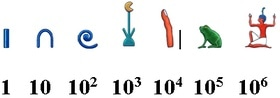Fig.1 Egyptian Hieroglyphic System (Additive numeral systems of Type 1)

Example: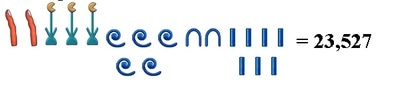The additive systems of Type 2 have special symbols for 1, 10, 100, 1000…. In addition, they have special symbols for 5, 50, 500…. The Roman numerals belong to additive systems of Type 2 (see Fig.2):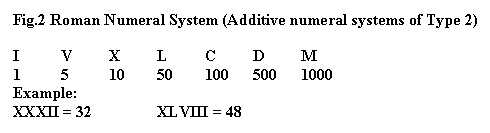The additive systems of Type 3 use alphabets to represent the numbers.

The example of such a system would be Greek alphabetic number system (see Fig.3):

Fig.3 Greek Alphabetic Numeral System (Additive numeral systems of Type 3)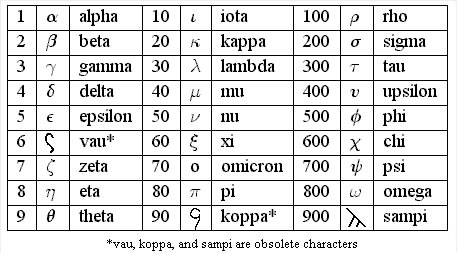In alphabetic numeral system, each letter has a numeric value.

Then, any number can be represented by the set of letters, using the additive principle.

For instance,

12 = ?Ã

543 = fÂµ?

There is special notation for the numbers greater than 999.

Note that alphabetic numeral systems could appear only when the alphabets and the writing systems came to the scene. Before that, many civilizations, including Greeks had other numeral systems, mostly the additive systems of Type 1 or 2.

2. Hybrid numeral systems

The hybrid numeral systems are the systems that use the principles of addition and multiplication.

There are few types of hybrid numeral systems. The example is a common Chinese number system (see Fig.4):

Fig.4 Common Chinese Numeral System (Hybrid numeral system)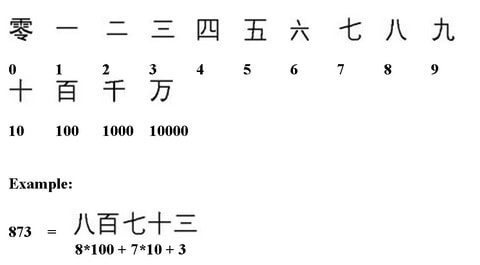Common Chinese numeral system has symbols for the numbers from 1 to 9 and for 10, 100, 1000 and 10000. It didn’t have a zero, but then later the symbol for zero was added.

3. Positional numeral systems

The difficulties of ancient additive and hybrid numeral systems are obvious:

– They didn’t have clear intuitive notation for the numbers.

– Representation of the big numbers usually required a lot of space

– Demand for the numbers greater than already existed in the system, required development of the new symbols or conventions.

– The additive and hybrid numeral systems were mostly static. Their number-symbols looked more like abbreviations and could appear only in the group of similar symbols or(and) in a certain position. That made the ancient numeral systems unsuitable for written arithmetic and requested auxiliary tools, like abacus, special tables or system-specific rules to do the calculations.

Therefore, the invention of positional numeral system was very important step for the human civilization.

In the positional system, the value of each symbol depends on position of that symbol in the number representation.

Except of our modern positional system, there were three positional systems in the history of civilization. They are systems of Babylonian scholars, Chinese scholars and Mayan astronomers.

The Babylonians used sexagesimal (base-60) positional numeral system, written in cuneiform (see Fig.5):

Fig.5 Babylonian sexagesimal positional numeral system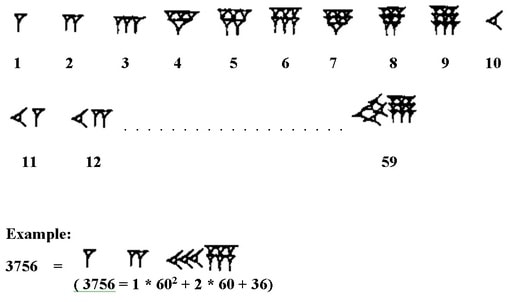The first positional systems had one significant drawback. They were not really dynamic.

Indeed, in order to be dynamic the positional system needs to have only one symbol (from available in that system) in each position. In Babylonian system, a digit in each position could be composed of a few repeated cuneiform symbols (in Chinese and Mayan numeral systems a digit could be composed of a few bars or points).

May be because of that drawback they couldn’t compete with the more advanced Hindu-Arabic positional numeral system.

Our modern decimal system, also called “Arabic” or “Hindu-Arabic”, has been invented in India; developed by Arabic mathematicians and then came to Europe.)

Today, the decimal system is widely accepted. It became an official numeral system in practically all countries.

Three more positional systems – binary, octal and hexadecimal became very important in the last 40-50 years with the appearance of computers and information technology.

The positional numeral systems have following main characteristics:

– Can represent practically any number

– Have special symbol zero that indicates the absence of the value (empty value) in the specific position

– The most suitable for the mathematical calculations among all numeral systems

– Provide the highest level of abstraction

### Numbers’ Conversion in Positional Numeral Systems

The positional systems have base (radix).

The base is the amount of unique symbols that positional numeral system uses for the numbers’ representation. For instance, base-10 (decimal) numeral system has ten symbols 0,1,2 ,3,4,5,6,7,8,9,10; base-2 (binary) numeral system has two symbols 0,1.

It is obvious that numeral systems with the higher base provide more compact notation for the numbers.

Indeed, the same number 25510 = 111111112 = FF16 will occupy 3 positions in decimal numeral system, 8 positions in binary numeral system and 2 positions in hexadecimal numeral system.

However, it doesn’t mean that we need to switch to base-100 or base-1000 numeral system. It is much easier for us to recognize and operate with 10 distinct symbols (may be because human being has 10 fingers), than with 100 or 1000.

At the same time for the computers, it is easier to operate with two figures, even though it may produce a long sequence of binary symbols for the very large numbers.

Theoretically, the amount of the positional numeral systems can be unlimited.

In practice, there is a few dozens of different positional numeral systems and often we need to convert the numbers from one system to another.

In this paragraph, we will discuss SQL techniques that can be used for the numbers conversion.

Let us start with the number conversion from base-n numeral system to base-10 numeral system, where radix n can be any radix different than 10.

As an example, let us convert the binary numbers into decimal ones.

First, create and load an auxiliary table that will store the sequence of whole numbers (see Listing1):

Listing1. Create and load an auxiliary table

SET NOCOUNT ON;
DECLARE @n INT;
SET @n = 100000;

IF EXISTS(SELECT * FROM sysobjects
WHERE ID = (OBJECT_ID(‘sequence’)) AND xtype = ‘U’)
DROP TABLE sequence;
CREATE TABLE sequence(num bigint NOT NULL PRIMARY KEY);

WITH numbers AS
(
SELECT 1 AS num
UNION ALL
SELECT num + 1 FROM numbers WHERE num < @n
)
INSERT INTO sequence
SELECT num FROM numbers
OPTION (MAXRECURSION 0);

Technique 1

Create and load test table (see Listing2):

Listing2. Create and load test table

SET NOCOUNT ON;
IF EXISTS(SELECT * FROM sysobjects
WHERE ID = (OBJECT_ID(‘baseN_Values’)) AND xtype = ‘U’)
DROP TABLE baseN_Values;

CREATE TABLE baseN_Values (baseN_Val VARCHAR(100));

INSERT INTO baseN_Values VALUES (‘10101’);
INSERT INTO baseN_Values VALUES (‘101010’);
INSERT INTO baseN_Values VALUES (‘10101000000000000001’);
INSERT INTO baseN_Values VALUES (‘101’);
INSERT INTO baseN_Values VALUES (‘1010’);
INSERT INTO baseN_Values VALUES (‘1010101’);
INSERT INTO baseN_Values VALUES (‘111111111’);
INSERT INTO baseN_Values VALUES (‘101011000100010001000100010010’);
INSERT INTO baseN_Values VALUES (‘1010101111111111’);
INSERT INTO baseN_Values VALUES(‘10101011111111111111111111111111111111111111111110’);
INSERT INTO baseN_Values VALUES (‘101010000000000000000000011’);
INSERT INTO baseN_Values VALUES (‘10101001010101010101’);
INSERT INTO baseN_Values VALUES (‘101010010101010101011111000’);
INSERT INTO baseN_Values VALUES (‘1010101000’);
INSERT INTO baseN_Values VALUES (‘101010000000000’);
INSERT INTO baseN_Values VALUES (‘10000000101010’);
INSERT INTO baseN_Values
VALUES (‘1000000000100000000000100000000000’);

Now, you need only one SELECT statement to convert the numbers (see Listing3):

Listing3. Convert numbers from base-n numeral system to decimal one

DECLARE @base bigint;
SET @base = 2;

SELECT t1.baseN_Val,
(SELECT SUM(SUBSTRING( t2.baseN_Val, LEN(t2.baseN_Val)-num + 1, 1)* POWER(@base, num -1))
FROM sequence CROSS JOIN baseN_Values t2
WHERE t1.baseN_Val = t2.baseN_Val
AND num <= LEN(t1.baseN_Val)) dec_Val
FROM baseN_Values t1;

In fact, SELECT statement from the Listing3 is a SQL notation of well-known formula that represents a real number X in the positional base-r numeral system:

X = cnRn + cn – 1Rn – 1 +…+ ciRi +… + c1R1 + c0R0 + c-1R-1 + … + c-(n-1)R-(n-1) + c-nR-n

, where coefficient-multiplier ci can be any symbol from the base-r numbers’ set and R0, R1, … Ri are the radix in the power of the position-number minus 1.

Pay attention to data type of variable @base. That data type is bigint and defines data type of the result of the expression POWER(@base, num -1).

It is sufficient to have bigint in order to convert a 50-digits binary number

101010111111111111111111111111111111111111111111102

into decimal number

75646399990988610.

However, if you set variable @base to 8, assuming that table baseN_Values stores octal values, the script from the Listing3 will give you an error: Arithmetic overflow error converting expression to data type bigint.

That means bigint data type doesn’t have enough capacity to convert 50-digits octal number into decimal one. In order to convert a long octal number, you will need to make a change in the Listing3: you need to replace bigint data type of variable @base by float data type.

Using script from the Listing3, you can convert the numbers from any positional numeral system to decimal one.

Technique 2

Another approach to the numbers’ conversion is to build tables that specify the correspondence between the numbers in different numeral systems.

This approach can be easily implemented in SQL, using the following idea:

1. Load n unique symbols of the base-n numeral system into the table
2. Join that table to itself as many times, as the maximum number of digits you want to get.

For example, if you want to get 6-digits binary numbers, you can do this (see Listing4):

Listing4. Build sequence of 6-digits binary numbers

IF EXISTS(SELECT * FROM sysobjects
WHERE ID = (OBJECT_ID(‘Bin’)) AND xtype = ‘U’)
DROP TABLE Bin;

CREATE TABLE bin(binNum VARCHAR(100));
INSERT INTO bin VALUES(‘0’);
INSERT INTO bin VALUES(‘1’);

SELECT (b1.binNum + b2.binNum + b3.binNum +
b4.binNum + b5.binNum + b6.binNum) AS binValue
FROM bin b1 CROSS JOIN bin b2 CROSS JOIN bin b3
CROSS JOIN bin b4 CROSS JOIN bin b5 CROSS JOIN bin b6
ORDER  BY 1

The query from the Listing4, however, is very inconvenient. The problem is in its static nature.

Indeed, for 6-digits binary numbers you need 6 joins and 6 addends in the SELECT list. For 10-digits binary numbers you would need 10 joins and 10 addends in the SELECT list.

Each time when you need a specific length of the numbers, you need to rewrite the query.

You can solve that problem using dynamic SQL, but in SQL Server 2005 you can do something more elegant (see Listing5):

Listing5. Build sequence of 10-digits binary numbers using CTE

IF EXISTS(SELECT * FROM sysobjects
WHERE ID = (OBJECT_ID(‘Bin’)) AND xtype = ‘U’)
DROP TABLE Bin;

CREATE TABLE bin(binNum VARCHAR(100));
INSERT INTO bin VALUES(‘0’);
INSERT INTO bin VALUES(‘1’);

DECLARE @lenght INT;
SELECT @lenght = 10;

WITH numbers AS

SELECT CAST(binNum AS VARCHAR(100)) AS base2 FROM bin
UNION ALL
SELECT CAST((t2.binNum + base2) AS VARCHAR(100))
FROM numbers CROSS JOIN bin t2
WHERE LEN(base2) < @lenght

SELECT ROW_NUMBER() OVER (ORDER BY base2) – 1 AS base10, base2
FROM numbers
WHERE LEN(base2) > @lenght – 1
OPTION (MAXRECURSION 0);

This solution will change the query dynamically, but it works only for binary numbers.

If you want your solution to work with any base (well, almost any), you need to use the approach, shown in the Listing6:

Listing6. Almost universal solution with user-defined function

IF OBJECT_ID (N’dbo.udf_GetNumbers’, N’TF’) IS NOT NULL
DROP FUNCTION dbo.udf_GetNumbers;
GO

CREATE FUNCTION dbo.udf_GetNumbers (@base INT, @lenght INT)
RETURNS @baseN_numbers TABLE
(
decNum INT PRIMARY KEY NOT NULL,
baseN_Num VARCHAR(50) NOT NULL
)
AS
BEGIN
WITH tblBase AS
(
SELECT CAST(0 AS VARCHAR(50)) AS baseNum
UNION ALL
SELECT CAST((baseNum + 1) AS VARCHAR(50))
FROM tblBase WHERE baseNum < @base-1
),
numbers AS
(
SELECT CAST(baseNum AS VARCHAR(50)) AS num FROM tblBase
UNION ALL
SELECT CAST((t2.baseNum + num) AS VARCHAR(50)) FROM numbers CROSS JOIN tblBase t2
WHERE LEN(NUM) < @lenght
)
INSERT INTO @baseN_numbers
SELECT ROW_NUMBER() OVER (ORDER BY NUM) -1 AS rowID, NUM
FROM numbers WHERE LEN(NUM) > @lenght – 1
OPTION (MAXRECURSION 0);
RETURN
END

From here, everything becomes easy. If you need, for example to build a table with decimal and corresponding binary, ternary and octal numbers, you can run the next query (see Listing7):

Listing7. Example with decimal, binary, ternary and octal numbers

SELECT U1.decNum base10,
U1.baseN_Num base2,
U2.baseN_Num base3,
U3.baseN_Num base8
FROM dbo.udf_GetNumbers(2, 10) U1
INNER JOIN dbo.udf_GetNumbers(3, 7) U2 ON u1.decNum = u2.decNum
INNER JOIN dbo.udf_GetNumbers(8, 4) U3 ON u2.decNum = u3.decNum

As I mentioned earlier, the solution from the Listing6 is almost universal. It will work fine for all the bases lower than 10.

However, for any numeral system, that has special symbols (like A and B in duodecimal numeral system) you will need explicitly load table with all the symbols of that base-n system and then run the query from the Listing5.

### Conversion from Decimal to Roman Numbers in SQL

Roman numeral system (adapted from Etruscans numeral system) was in use in ancient Rome and Roman Empire for more than thousand years.

That system was the main numeral system in Europe until 14th century and then gradually, during the centuries was supplanted by the Hindu-Arabic decimals.

Today, Roman numerals are still in use. They can be found in clocks and calendars; in books and media; in art, antique shops and museums; in chemistry; as the list numbers (you can enumerate pages of the Word document, using the roman numerals) and more.

As we saw earlier, the Roman numerals belong to additive numeral systems (see Fig2). Decimal numbers in turn represent positional numeral systems.

Both systems are governed by different rules and that makes the numbers’ conversion between Roman and decimal systems quite tricky.

However, there is a simple way to do such a conversion in SQL.

First, create auxiliary tables with Roman numbers (see Listing8):

Listing8. Create auxiliary tables with Roman numbers

/* Basic symbols of Roman numerals

I  V   X   L   C   D   M
1  5   10  50  100 500 1000

——————————————*/

IF EXISTS(SELECT * FROM sysobjects
WHERE ID = (OBJECT_ID(‘dec_rom1_9’)) AND xtype = ‘U’)
DROP TABLE dec_rom1_9;
GO
SELECT t1.decNum, t1.romaNum INTO dec_rom1_9
FROM
(SELECT 0 AS decNum, ” AS romaNum
UNION ALL
SELECT 1, ‘I’
UNION ALL
SELECT 2, ‘II’
UNION ALL
SELECT 3, ‘III’
UNION ALL
SELECT 4, ‘IV’
UNION ALL
SELECT 5, ‘V’
UNION ALL
SELECT 6, ‘VI’
UNION ALL
SELECT 7, ‘VII’
UNION ALL
SELECT 8, ‘VIII’
UNION ALL
SELECT 9, ‘IX’
) t1
——————————————————–

IF EXISTS(SELECT * FROM sysobjects
WHERE ID = (OBJECT_ID(‘dec_romX_XC’)) AND xtype = ‘U’)
DROP TABLE dec_romX_XC;
GO
SELECT t1.decNum, t1.romaNum INTO dec_romX_XC
FROM
(SELECT 0 AS decNum, ” AS romaNum
UNION ALL
SELECT 10, ‘X’
UNION ALL
SELECT 20, ‘XX’
UNION ALL
SELECT 30, ‘XXX’
UNION ALL
SELECT 40, ‘XL’
UNION ALL
SELECT 90, ‘XC’
) t1
——————————————————–

IF EXISTS(SELECT * FROM sysobjects
WHERE ID = (OBJECT_ID(‘dec_romC_CM’)) AND xtype = ‘U’)
DROP TABLE dec_romC_CM;
GO
SELECT t1.decNum, t1.romaNum INTO dec_romC_CM
FROM
(SELECT 0  AS decNum, ” AS romaNum
UNION ALL
SELECT 100, ‘C’
UNION ALL
SELECT 200, ‘CC’
UNION ALL
SELECT 300, ‘CCC’
UNION ALL
SELECT 400, ‘CD’
UNION ALL
SELECT 900, ‘CM’
) t1

IF EXISTS(SELECT * FROM sysobjects
WHERE ID = (OBJECT_ID(‘dec_romM’)) AND xtype = ‘U’)
DROP TABLE dec_romM;
GO
SELECT t1.decNum, t1.romaNum INTO dec_romM
FROM
(SELECT 0  AS decNum, ” AS romaNum
UNION ALL
SELECT 1000, ‘M’
UNION ALL
SELECT 2000, ‘MM’
UNION ALL
SELECT 3000, ‘MMM’) t1

Now you can get the decimal numbers with the corresponding Roman numbers in one query (see Listing9):

Listing9. Get decimal and corresponding Roman numbers

SELECT (t1.decNum + t2.decNum) AS decNum,
(t1.romaNum + t2.romaNum) AS romaNum
FROM dec_romM t1 CROSS JOIN
(SELECT (t1.decNum + t2.decNum) AS decNum,
(t1.romaNum + t2.romaNum) AS romaNum
FROM (SELECT 0 AS decNum, ” AS romaNum
UNION ALL SELECT 500, ‘D’) t1
CROSS JOIN
(SELECT (t1.decNum + t2.decNum) AS decNum,
(t1.romaNum + t2.romaNum) AS romaNum
FROM dec_romC_CM t1 CROSS JOIN
(SELECT (t1.decNum + t2.decNum) AS decNum,
(t1.romaNum + t2.romaNum) AS romaNum
FROM (SELECT 0 AS decNum, ” AS romaNum
UNION ALL SELECT 50, ‘L’) t1
CROSS JOIN
(SELECT (t1.decNum + t2.decNum) AS decNum,
(t1.romaNum + t2.romaNum) AS romaNum
FROM dec_romX_XC t1 CROSS JOIN dec_rom1_9 t2) t2
WHERE NOT (t1.romaNum = ‘L’ AND t2.romaNum LIKE (‘%[LC]%’))) t2) t2
WHERE NOT (t1.romaNum = ‘D’ AND t2.romaNum LIKE (‘%[DM]%’))) t2
ORDER BY 1

For the basic symbols equal or greater than 4000, the horizontal bar should be placed on top of that symbol. That horizontal bar indicates multiplication by 1000. However, ASCII doesn’t have the Roman symbols with horizontal bar on top. This is why the solution from the Listing9 shows the Roman numbers up to 4000 only.

Practically, you can convert the numbers from almost any numeral system to modern one, using SQL. However, to do that, you need to find the character set with the required basic symbols of that numeral system.

### Historical Puzzle

It is widely accepted as an axiom that Hindu/Arabic numeral system is much better and more advanced than Roman numeral system.

The main arguments are:

• Roman numerals don’t have zero.
• Roman numbers occupy more space than decimal numbers.
• The arithmetic calculations with Roman numbers are very complicated or even impossible.
• Roman numbers cannot be used in abstract mathematics.

Indeed, Romans don’t have as many achievements in pure mathematics as ancient Greeks. They were more interested in practical mathematics in order to build the roads, bridges and other constructions. They developed a special abacus (tabula) to facilitate the mathematical calculations.

However, even though Hindu/Arabic numeral system was accepted in Islamic Spain in 9th century, it took another 600 – 700 years to make it dominant number system in whole Europe.

During those 600-700 years all the disputes between Abacists (devotees of Roman numeral system) and Algorists (supporters of decimal numeral system) ended with the same result – nobody could prove the advantage of their system.

There are a few explanations why Hindu/Arabic positional system finally won:

• It was much more convenient and faster for merchants and bookkeepers to use decimal numbers.
• Hindu/Arabic system was more suitable for printing.
• The book “Liber Abaci“of Leonardo Fibonacci helped to spread decimal positional system in Europe.
• Roman numeral system restricted progress of mathematics and so development of civilization.

However, even now some people wondering whether it is possible to do the arithmetic calculations with roman numerals without converting them to an Arabic/Hindu notation.

If you know such a method, I’d be fascinated to know how it was done, especially in TSQL.

The editor will give a prize for the best SQL program that makes possible the addition (subtraction) operations with Roman numerals without conversion.